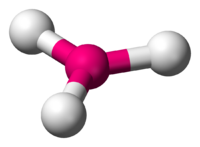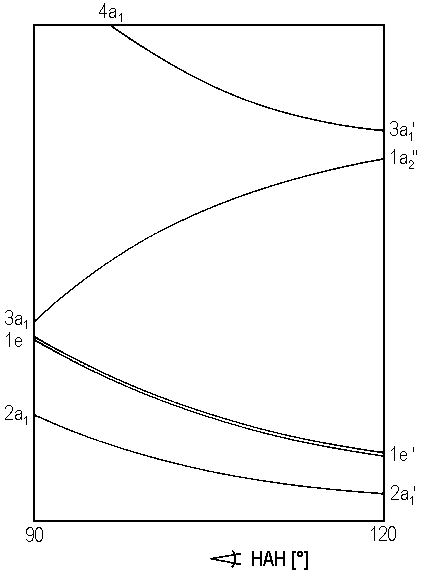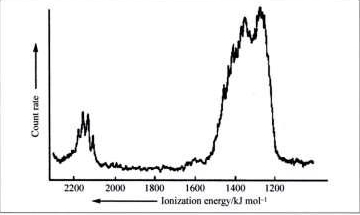# Homework 3

Name: ______________________________

Section: _____________________________

Student ID#:__________________________

## Q1

For the following molecules identify, use the Walsh diagram for bending of $$AH_2$$ molecules to predict whether the molecule will be linear or bent and if so, what the electronic configuration is (note the notation for the molecular orbitals of linear and bent molecules differ). LiH2+ , BeH2+ ,BeH2, BH2+, BH2, 3CH2, 1CH2, NH2 ,OH2. If NeH2 were a valid molecule, what geometry would you expect it would have?

## Q2

The conformation of a molecule $$AH_3$$ can be predicted with a Walsh's diagram shown below.Trigonal pyramidal (left) vs. Trigonal planar (right)

Note that an angle HAH of 120° indicates a trigonal planar geometry, whereas angles below 120° represents a trigonal pyramidal geometry.1. Predicted the geometry and electronic configuration (not different notation for planar and pyramidal geometries) of the following species: BH3, CH3+ CH3, NH3 and OH3+.
2. The difference between the equilibrium energy and the lowest energy for a planar state of the molecule is the inversion barrier (example for ammonia is shown below). Which species do you think as a higher inversion barrier and why: CH3 or CH3?## Q3

For the below photoelectron spectrum of neon, each band corresponds to the binding energies of the electron in occupied atomic orbitals. Label each band to the corresponding atomic orbitals in neon.## Q4

Diagram the expected photoelectron spectrum for the nitrogen and chlorine diatomics. Assume the incident photon energy is sufficient to ionize only valence electrons (e.g., ignore the core electrons). Which would you expect to have the lower possible ionization energy (outline each factor that is involved in this estimate).

## Q5

What does the photoelectron spectrum of methane say about the nature of the $$sp^3$$ hybridization in methane?## Q6

Explain using an energy level molecular orbital diagram whether $$N_2$$ or $$N_2^–$$ has a longer bond and why.

## Q7

Explain using energy level molecular orbital diagrams why $$O_2$$ and $$B_2$$ are paramagnetic while all of the other first row homonuclear diatomic molecules are diamagnetic.